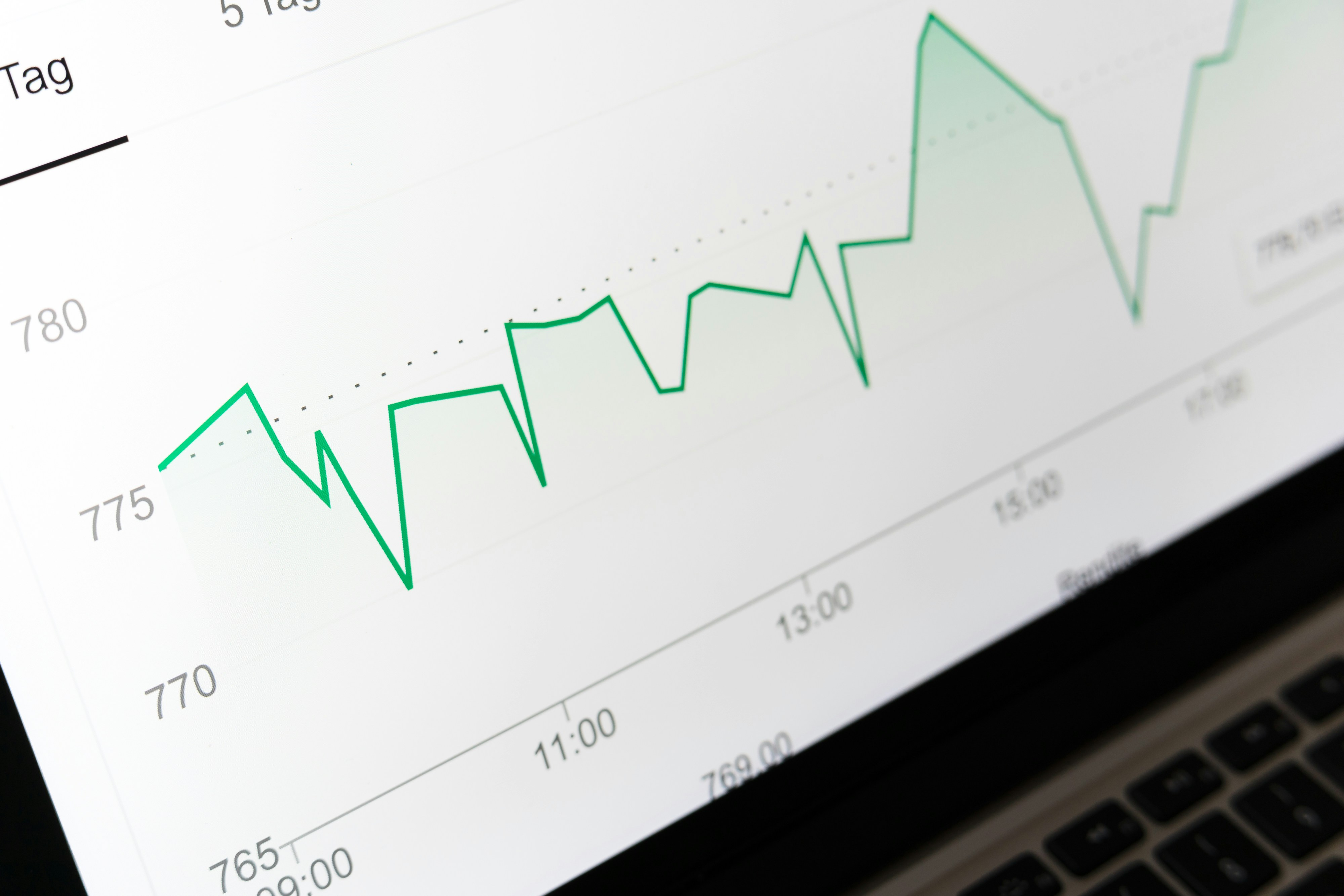Mahmoud Ahmed

Postdoc - Biomedicine

# Introduction to data analysis in R

A gentle introduction to data analysis in R

### Description

This is a short course on data analysis in R. The goal is to get the participants familiar with R code and to appreciate it as a tool to handle common experimental data. The materials are delivered in the form of transitional lectures and interactive exercises.

### Objectives

• To get familiar with R as a tool for data analysis
• To apply basic arithmetic and statistics in R
• To learn to handle experimental data in R (qPCR and images)

### Format

Each module is delivered in three steps

• `lectures/`: slides discussing the main concepts
• `practice/`: interactive exercises for practicing purposes
• `homework/`: more exercises to test understanding

### Outline

• Getting started in R
• Lecture ([Slides](lectures/lecture_1.pdf))
• Basic statistics in R
• Lecture ([Slides](lectures/lecture_2.pdf))
• Quantifying mRNA using the `pcr` package
• Lecture ([Slides](lectures/lecture_3.pdf))
• Quantifying protein co-localization in fluorescence images using the `colocr` package